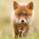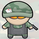צפיות ‎1175‎
grid based on the cumulative average price.

on a sidenote: looks like im baned from chat for a week.
```study("[RS]CAP Grid", overlay=true)

c = cum(close)/(1+n)
h = cum(high)/(1+n)
l = cum(low)/(1+n)

factor = h - l

plot(c, color=black, linewidth=2)
plot(h, color=black, linewidth=2)
plot(h + factor, color=black, linewidth=2)
plot(h + factor * 2, color=black, linewidth=2)
plot(h + factor * 3, color=black, linewidth=2)
plot(h + factor * 4, color=black, linewidth=2)
plot(h + factor * 5, color=black, linewidth=2)

plot(l, color=black, linewidth=2)
plot(l - factor, color=black, linewidth=2)
plot(l - factor * 2, color=black, linewidth=2)
plot(l - factor * 3, color=black, linewidth=2)
plot(l - factor * 4, color=black, linewidth=2)
plot(l - factor * 5, color=black, linewidth=2)
```

## תגובותInteresting approach! Have you tried using as "factor multipliers" fibonacci/padovan progressions..? ;)
השבxel_arjona
possibly, likely unpublished, just a matter of changing the multipliers.
the padovan sequence looks interesting, 1st time i heard of it :p
השבRicardoSantos
When you help me to learn pine when writing my "Fractal Bands", we wrote the algorithm to calculate mathematically both of this progressions in pine. (recursive innecesary but fur fun! ) :D
// N Root Function
nroot(index,number) =>
cond_r = index == 0 or number == 0 or number < 0
If_True = 0
If_False = (exp((1/index) * log(number)))
iff(cond_r, If_True, If_False)
//Algebraic Constants for general calculations.
_phi = (1+sqrt(5))/2 // Phi Number (Fibonacci Seq.)
_pn = (nroot(3,(108 + 12*sqrt(69))) + nroot(3,(108 - 12*sqrt(69))))/6 // Plastic Number (Podovan Seq.)
השבxel_arjona
aw i see, i remember the fib number function :p
השבRicardo, interesting indicator.
I coded an EMA with ATR bands that serves a similar purpose.

Can I pm you with a request?
I'm always impressed by your coding skills.
השבI'm curious. Why does the distance between central lines are shorter than others?
השבalex.a
they are the respective lines for the cumulative average of high close and low.
השבRicardoSantos
I should I interpret that, if the price was in that range, usually the distance between H and L were shorter???
השבalex.a
in my opinion it would mean its at its normal price, end/start of cycle
השב